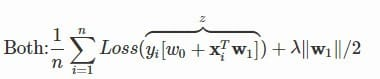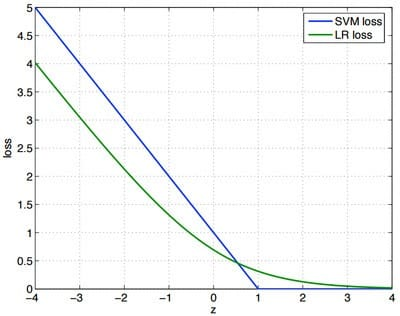# 损失函数

4/14/2021 损失函数θ

## # 请说说你对逻辑回归损失函数的理解

Log损失函数的标准形式：

logistic回归的表达式如下（为了将类别标签y统一为1和0，下面将表达式分开表示）： $$P\left(Y=y^{\left(i\right)}|x^{\left(i\right)};\theta\right)=\left{\begin{array}{l} h_{\theta}\left(x^{\left(i\right)}\right)=\frac{1}{1+e^{-\theta^Tx}},,,y^{\left(i\right)}=1\ 1-h_{\theta}\left(x^{\left(i\right)}\right)=\frac{e^{-\theta^Tx}}{1+e^{-\theta^Tx}},,,y^{\left(i\right)}=0\ \end{array}\right.$$ 将上面的公式合并在一起，可得到第个样本正确预测的概率：

## # 请说说你对平方损失函数的理解。

• 简单，计算方便；
• 欧氏距离是一种很好的相似性度量标准；
• 在不同的表示域变换后特征性质不变。

## # 请你对逻辑斯谛回归和SVM的损失函数进行一下对比。这两个损失函数的目的都是增加对分类影响较大的数据点的权重，减少与分类关系较小的数据点的权重。SVM的处理方法是只考虑support vectors，也就是和分类最相关的少数点，去学习分类器。而逻辑回归通过非线性映射，大大减小了离分类平面较远的点的权重，相对提升了与分类最相关的数据点的权重,两者的根本目的都是一样的。

svm考虑局部（支持向量），而logistic回归考虑全局，就像大学里的辅导员和教师间的区别。

1. LR采用log损失，SVM采用合页损失。
• LR对异常值敏感，SVM对异常值不敏感。
• 在训练集较小时，SVM较适用，而LR需要较多的样本。
• LR模型找到的那个超平面，是尽量让所有点都远离他，而SVM寻找的那个超平面，是只让最靠近中间分割线的那些点尽量远离，即只用到那些支持向量的样本。
• 对非线性问题的处理方式不同，LR主要靠特征构造，必须组合交叉特征，特征离散化。SVM也可以这样，还可以通过kernel。
• svm 更多的属于非参数模型，而logistic regression 是参数模型，本质不同。其区别就可以参考参数模型和非参模型的区别

1. 如果Feature的数量很大，跟样本数量差不多，这时候选用LR或者是Linear Kernel的SVM
2. 如果Feature的数量比较小，样本数量一般，不算大也不算小，选用SVM+Gaussian Kernel
3. 如果Feature的数量比较小，而样本数量很多，需要手工添加一些feature变成第一种情况。(LR和不带核函数的SVM比较类似。)

## # 对于逻辑回归，为什么说平方损失函数是非凸的？

https://www.zhihu.com/question/264396874

## # 如何把SVM的推导和损失函数联系起来？

https://www.zhihu.com/question/62881491

## # softmax和cross-entropy是什么关系？

https://www.zhihu.com/question/294679135

## # 神经网络的损失函数为什么是非凸的?

https://www.zhihu.com/question/265516791